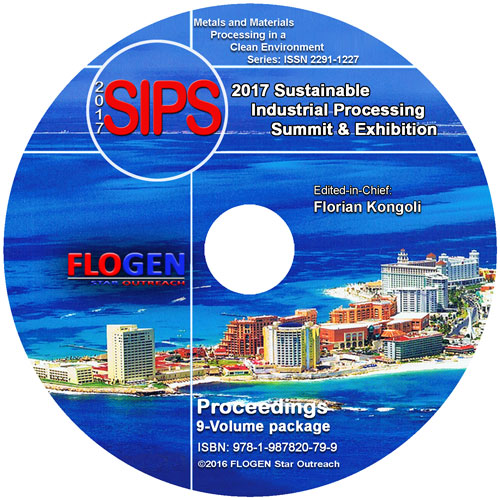## 2017-Sustainable Industrial Processing SummitSIPS 2017 Volume 6. Mathematics, Multiscale Mechanics, Coatings

 Editors: Kongoli F, Masset P, Rokicki P Publisher: Flogen Star OUTREACH Publication Year: 2017 Pages: 142 pages ISBN: 978-1-987820-71-3 ISSN: 2291-1227 (Metals and Materials Processing in a Clean Environment Series)CD shopping page

## On the Negative-Energy 4-Spinors and Masses in the Dirac Equation

Valeriy Dvoeglazov1;
Type of Paper: Regular
Id Paper: 36
Topic: 38

## Abstract:

It is easy to check that both algebraic equation $Det (hat p - m) =0$ and $Det (hat p + m) =0$ for $u-$ and $v-$ 4-spinors have solutions with $p_0= pm E_p =pm sqrt{{bf p}^2 +m^2}$. The same is true for higher-spin equations. Meanwhile, every book considers
the equality $p_0=E_p$ for both $u-$ and $v-$ spinors of the $(1/2,0)oplus (0,1/2))$ representation only, thus applying the Dirac-Feynman-Stueckelberg procedure for elimination of the negative-energy solutions. The recent Ziino works (and, independently,
the articles of several others) show that the Fock space can be doubled. We re-consider this possibility on the quantum field level for both $s=1/2$ and higher spin particles.

## Keywords:

cosmology; hadronic mechanics; physics; quantum mechanics;